# uitreenode

Create tree node component

## Syntax

``node = uitreenode``
``node = uitreenode(parent)``
``node = uitreenode(parent,sibling)``
``node = uitreenode(parent,sibling,location)``
``node = uitreenode(___,Name,Value)``

## Description

````node = uitreenode` creates a tree node UI component inside a tree within a new figure window and returns the `TreeNode` object. MATLAB® calls the `uifigure` function to create the figure.```
````node = uitreenode(parent)` creates a tree node in the specified parent container. The parent container can be a `Tree` or `TreeNode` object.```
````node = uitreenode(parent,sibling)` creates a tree node in the specified parent container, after the specified sibling node.```

example

````node = uitreenode(parent,sibling,location)` creates a tree node, and places it after or before the sibling node. Specify `location` as `"after"` or `"before"`.```

example

````node = uitreenode(___,Name,Value)` specifies `TreeNode` property values using one or more `Name,Value` pair arguments. Specify `Name,Value` as the last set of arguments when you use any of the previous syntaxes.```

## Examples

collapse all

Create a tree that contains a parent node called ```Sample Data``` with a child node called `Sample 1`. Expand the tree to see both nodes.

```fig = uifigure; t = uitree(fig); parent = uitreenode(t,"Text","Sample Data"); child = uitreenode(parent,"Text","Sample 1"); expand(t)```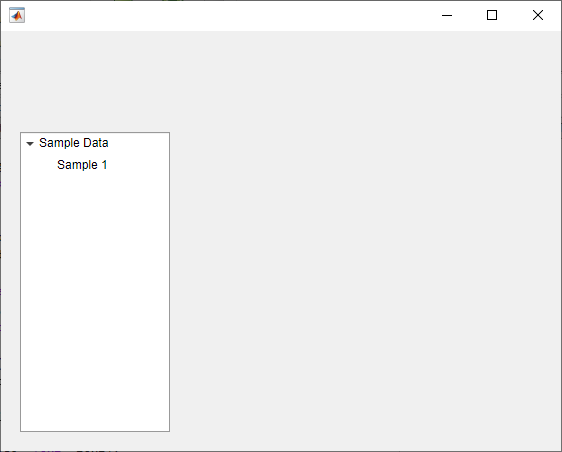Create a tree with a parent and child node. Expand the tree to see both nodes.

```fig = uifigure; t = uitree; p = uitreenode(t,"Text","Teas"); c1 = uitreenode(p,"Text","Green Tea"); expand(t);```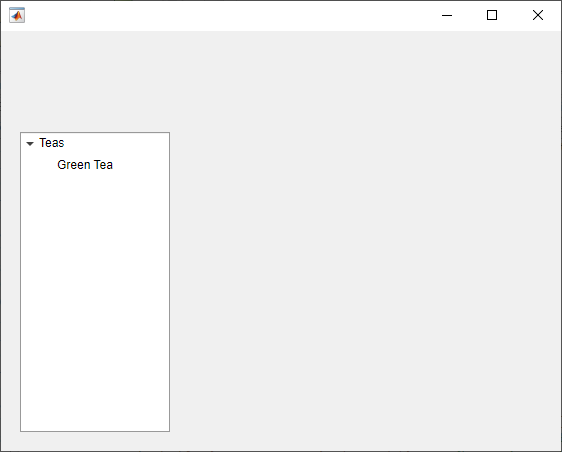Add a new child node to the tree above the `c1` node.

`c2 = uitreenode(p,c1,"before","Text","Oolong Tea");`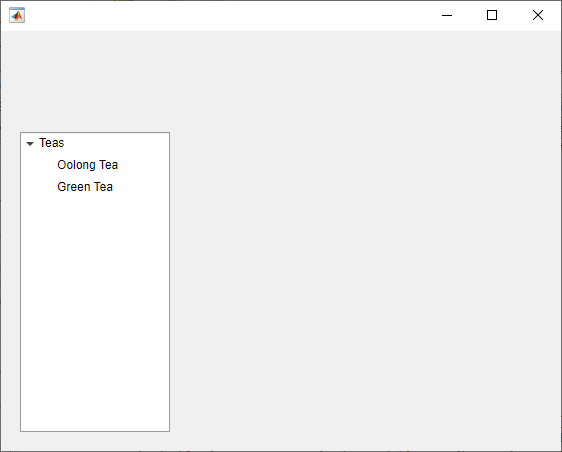Create an app that displays athlete names grouped by sport. When the app user clicks on a name, MATLAB displays data about the athlete.

Create a program file called `mytreeapp.m` that contains the following commands to create a tree, a set of nested tree nodes, and a callback function for the tree. The `SelectionChangedFcn` property specifies the function to execute when the user clicks a node in the tree.

```function mytreeapp fig = uifigure; t = uitree(fig,"Position",[20 20 150 150]); % Assign callback in response to node selection t.SelectionChangedFcn = @nodechange; % First level nodes category1 = uitreenode(t,"Text","Runners","NodeData",[]); category2 = uitreenode(t,"Text","Cyclists","NodeData",[]); % Second level nodes. % Node data is age (y), height (m), weight (kg) p1 = uitreenode(category1,"Text","Joe","NodeData",[40 1.67 58] ); p2 = uitreenode(category1,"Text","Linda","NodeData",[49 1.83 90]); p3 = uitreenode(category2,"Text","Rajeev","NodeData",[25 1.47 53]); p4 = uitreenode(category2,"Text","Anne","NodeData",[88 1.92 100]); % Expand the tree expand(t); % Create the function for the SelectionChangedFcn callback % When the function is executed, it displays the data of the selected item function nodechange(src,event) node = event.SelectedNodes; display(node.NodeData); end end```

When the user runs `mytreeapp` and clicks a node in the tree, MATLAB displays the `NodeData` for that node.Create a tree that populates nodes based on the data in a table.

Create a figure with a grid layout manager to hold the UI components. Load sample data on electric utility outages and create a table UI component to display the data. Then, create a tree to hold nodes listing the regions and causes of the outages.

```fig = uifigure; gl = uigridlayout(fig,[1 2]); gl.ColumnWidth = {'2x','1x'}; T = readtable("outages.csv"); T = T(1:20,["Region","OutageTime","Loss","Cause"]); tbl = uitable(gl,"Data",T); tr = uitree(gl);```

Specify the table variables to display in the tree. For each of those variables, create a top-level node whose text is the variable name. Extract the relevant data by converting the table entries for the variable to a categorical array and returning the list of categories as `names`. Then, loop through the categories. For each element, add a node to the tree under the appropriate parent node.

```vars = ["Region","Cause"]; for k1 = 1:length(vars) var = vars{k1}; varnode = uitreenode(tr,"Text",var); rows = T{:,var}; names = categories(categorical(rows)); for k2 = 1:length(names) text = names{k2}; uitreenode(varnode,"Text",text); end end```

Expand the tree to see all the nodes.

`expand(tr)`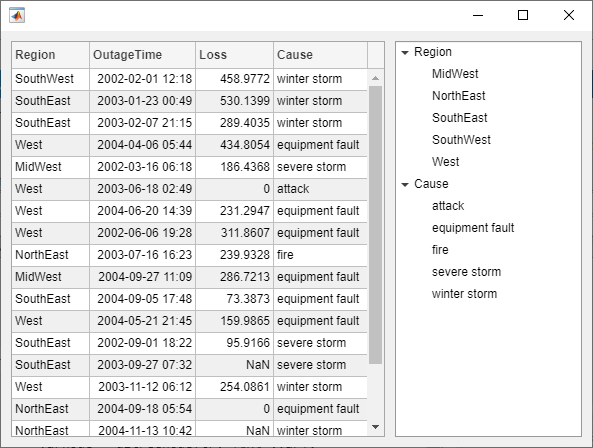Style nodes in a tree that showcases a file structure to visually distinguish different file types.

Create a tree UI component. Each top-level node represents a folder. Each child node represents a file in that folder. Expand the tree to see all the nodes.

```fig = uifigure("Position",[300 300 350 400]); t = uitree(fig); % Parent nodes n1 = uitreenode(t,"Text","App 1"); n2 = uitreenode(t,"Text","App 2"); n3 = uitreenode(t,"Text","Images"); % Child nodes n11 = uitreenode(n1,"Text","myapp1.m"); n21 = uitreenode(n2,"Text","myapp2.m"); n22 = uitreenode(n2,"Text","app2callback.m"); n31 = uitreenode(n3,"Text","peppers.png"); expand(t)```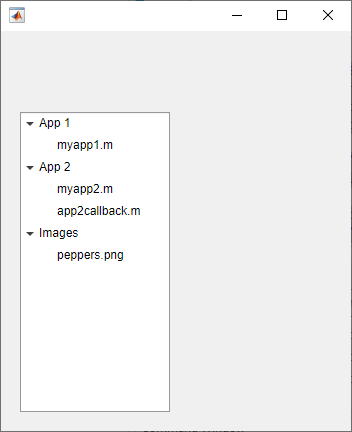Create three styles: one with a bold font weight, one with an italic font angle, and one with an icon.

```dirStyle = uistyle("FontWeight","bold"); mStyle = uistyle("FontAngle","italic"); imgStyle = uistyle("Icon","peppers.png");```

Apply the bold style to the top-level nodes to distinguish the nodes that represent folders. Apply the italic style to the children of the ```App 1``` and `App 2` nodes to distinguish the nodes that represent MATLAB program files. Finally, apply the icon style to the node that represents an image file to show a preview of the image.

```addStyle(t,dirStyle,"level",1) addStyle(t,mStyle,"node",[n1.Children;n2.Children]) addStyle(t,imgStyle,"node",n31)```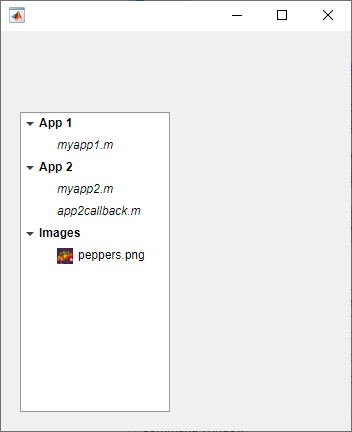Create a context menu for a tree component. Assign the context menu to all of the top-level nodes in the tree.

In a new script in your current folder, create a UI figure. Then, create a tree with four top-level nodes and a set of nested nodes.

```fig = uifigure; t = uitree(fig,"Position",[20 200 175 100]); category1 = uitreenode(t,"Text","Runners"); r1 = uitreenode(category1,"Text","Joe"); r2 = uitreenode(category1,"Text","Linda"); category2 = uitreenode(t,"Text","Cyclists"); c1 = uitreenode(category2,"Text","Rajeev"); category3 = uitreenode(t,"Text","Hikers"); h1 = uitreenode(category3,"Text","Jack"); category4 = uitreenode(t,"Text","Swimmers"); s1 = uitreenode(category4,"Text","Logan");```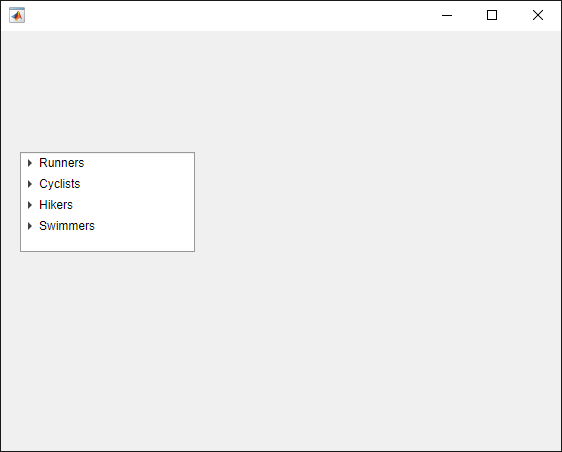Create a context menu with one menu item and two submenus that users can click to expand a single tree node or all of the tree nodes. For each submenu, specify a `MenuSelectedFcn` callback function to execute when a user selects the menu option. Pass the relevant app object as input to each function to access app data from within the callback function.

```cm = uicontextmenu(fig); m1 = uimenu(cm,"Text","Expand..."); sbm1 = uimenu(m1,"Text","This Node", ... "MenuSelectedFcn",{@expandSingle,fig}); sbm2 = uimenu(m1,"Text","All Nodes", ... "MenuSelectedFcn",{@expandAll,t});```

Assign the context menu to the top-level tree nodes by setting the `ContextMenu` property of each node to the `ContextMenu` object.

```category1.ContextMenu = cm; category2.ContextMenu = cm; category3.ContextMenu = cm; category4.ContextMenu = cm;```

At the bottom of the file, define the `expandSingle` and `expandAll` callback functions. Define the functions to accept the source and event data that MATLAB passes to all callback functions.

Define the `expandSingle` function to also accept the UI figure object containing the tree, and use the `CurrentObject` property of the figure to determine which tree node was clicked to bring up the context menu. Then, expand that node.

Define the `expandAll` function to also accept the tree object, and expand all of the nodes in the tree.

```function expandSingle(src,event,f) node = f.CurrentObject; expand(node) end function expandAll(src,event,t) expand(t) end```

Save and run the script. Right-click any of the top-level tree nodes to view the context menu.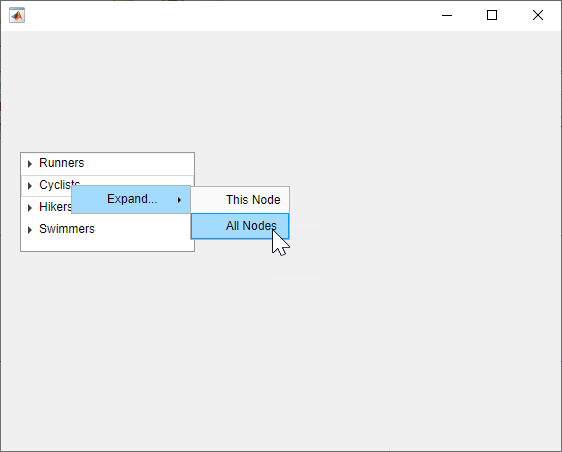## Input Arguments

collapse all

Parent object, specified as a `Tree` or `TreeNode` object.

Sibling node, specified as a `TreeNode` object.

Location of the node relative to its sibling, specified as `"after"` or `"before"`.

### Name-Value Arguments

Specify optional pairs of arguments as `Name1=Value1,...,NameN=ValueN`, where `Name` is the argument name and `Value` is the corresponding value. Name-value arguments must appear after other arguments, but the order of the pairs does not matter.

Example: `node = uitreenode(t,Text="Measurements")` creates a tree node with the label `"Measurements"`.

Before R2021a, use commas to separate each name and value, and enclose `Name` in quotes.

Example: `node = uitreenode(t,"Text","Measurements")` creates a tree node with the label `"Measurements"`.

Note

The properties listed here are a subset of the available properties. For a full list, see TreeNode Properties.

Node text, specified as a character vector or string scalar.

Icon source or file, specified as a character vector, a string scalar, or an `m`-by-`n`-by-3 truecolor image array. If you specify a file name, it can be an image file name on the MATLAB path or a full path to an image file. If you plan to share an app with others, put the image file on the MATLAB path to facilitate app packaging.

Supported image formats include JPEG, PNG, GIF, SVG, or `m`-by-`n`-by-3 truecolor image array. For more information about truecolor image arrays, see Image Types.

Example: `'icon.png'` specifies an icon file on the MATLAB path.

Example: `'C:\Documents\icon.png'` specifies a full path to an image file.

Node data, specified as an array of any type. Specify `NodeData` to share node-relevant data within your app code.

## Version History

Introduced in R2017b